Function Repository Resource:

Torus

Graphics3D primitives for a torus

Contributed by: Jon McLoone
 ResourceFunction["Torus"][] represents a unit radius torus centered at {0,0,0} with a primary radius of 1 and secondary radius of 1/2. ResourceFunction["Torus"][{x,y,z}] represents a unit radius torus centered at {x,y,z} with a primary radius of 1 and secondary radius of 1/2. ResourceFunction["Torus"][pos,{r1,r2}] represents a unit radius torus centered at pos with a primary radius of r1 and secondary radius of r2. ResourceFunction["Torus"][pos,{r1,r2},{ax,ay,az}] represents a unit radius torus centered at pos oriented around a center of rotation with direction {ax,ay,az}.

Examples

Basic Examples (2)

A unit torus:

 In:=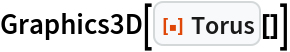Out=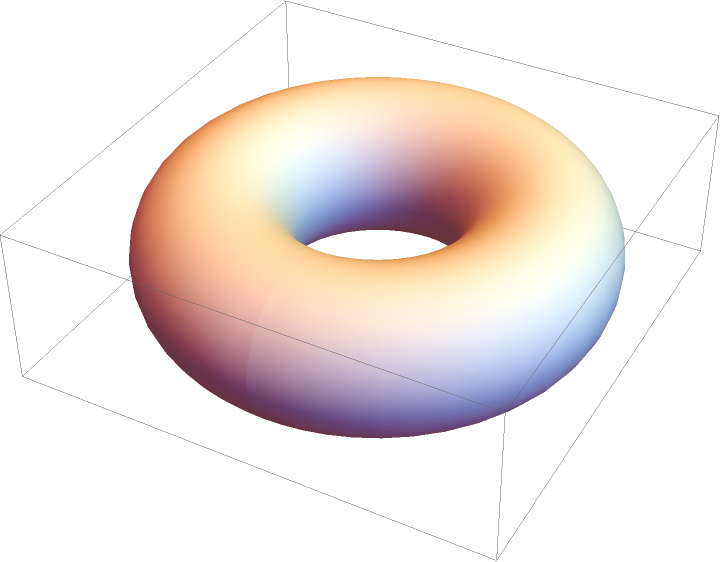A torus at {10,20,30} with primary radius of 20 and secondary radius of 1 around an orientation vector of {0,1,0}:

 In:=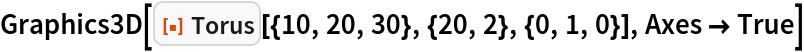Out=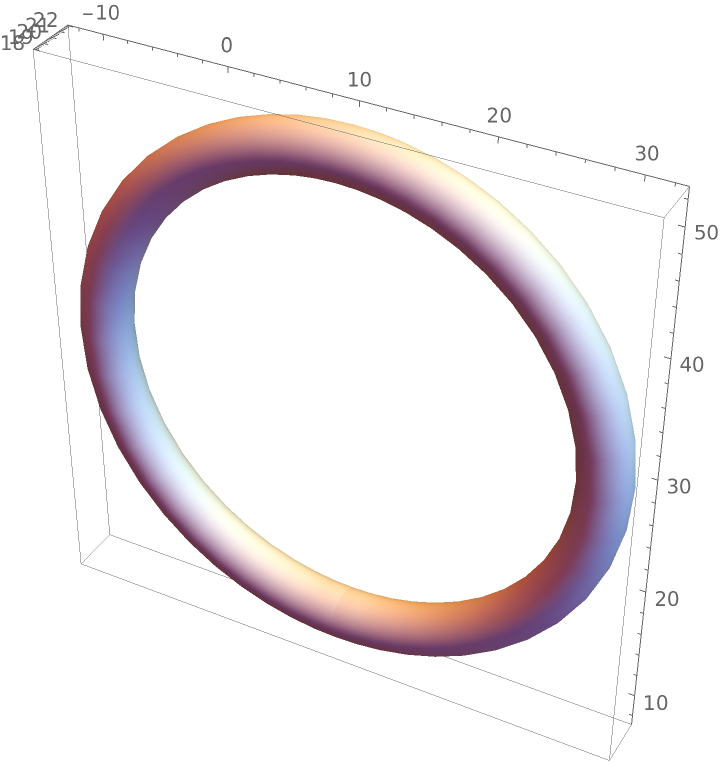Neat Examples

 In:=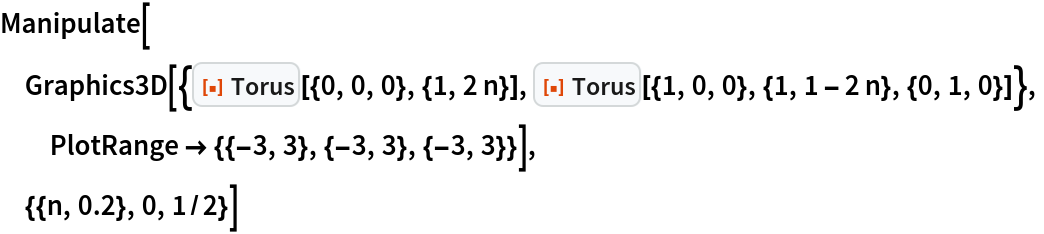Out=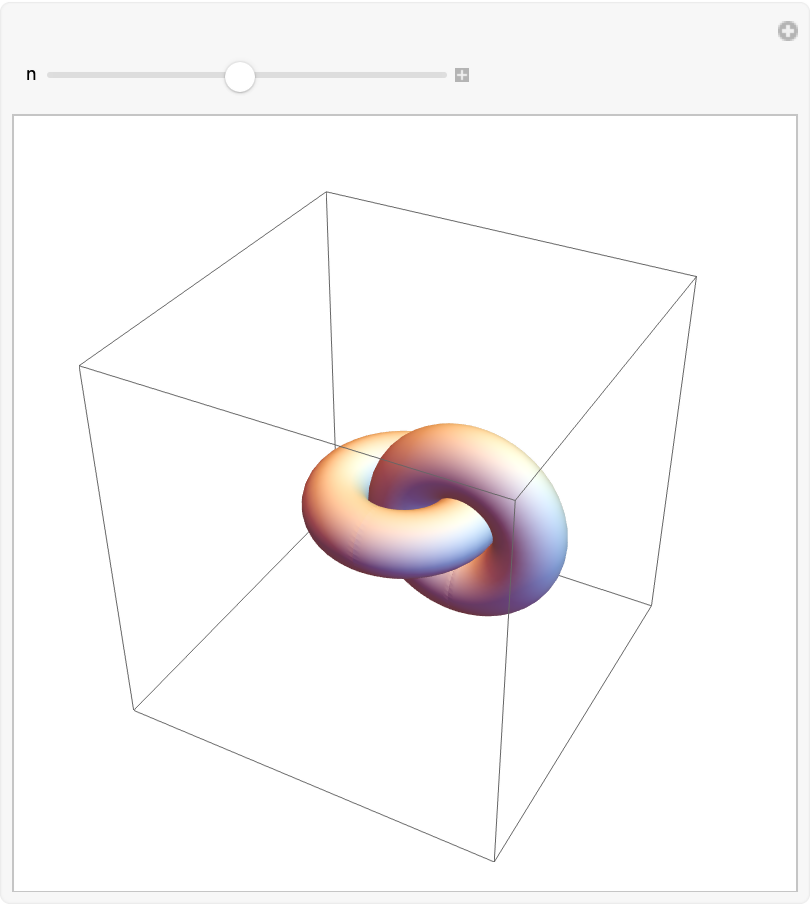Author Notes

Inner radius was changed from version one to fix a bug.